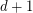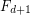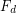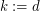Importance: High ✭✭✭
 Author(s): Krajicek, Jan
 Subject: Logic
 Keywords: Frege system short proof
 Posted by: zitterbewegung on: October 18th, 2007
Problem   Find a constantsuch that for anythere is a sequence of tautologies of depththat have polynomial (or quasi-polynomial) size proofs in depthFrege systembut requires exponential sizeproofs.

Such tautologies are known ifmay depend on(for). This problem is also closely related to conservativity relations among bounded arithmetic theories.

## Bibliography

*[K1] J.Krajicek: "Lower Bounds to the Size of Constant-Depth Propositional Proofs", J. of Symbolic Logic, 59(1), (1994), pp.73-86

[K2] J. Krajicek: "Bounded arithmetic, propositional logic, and complexity theory", Encyclopedia of Mathematics and Its Applications, Vol.60, Cambridge University Press, Cambridge - New York - Melbourne, (1995), 343 p. (on page 243)

* indicates original appearance(s) of problem.